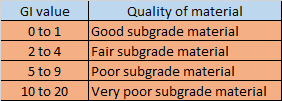## Translate

### CONJUGATE BEAM METHOD

Conjugate beam method is one of the best method of deflection which is used to find deflection of beams.

## Important facts about conjugate beam method

• Conjugate beam method is only used to find deflection due to bending forces applied on structure. This means that conjugate beam method cannot be used to find deflection due to shear, torsion, axial force, temperature change, etc.
• For beams having non-prismatic cross-section, the slope and deflection can be easily obtained by conjugate beam method.
• Conjugate beam method is very much helpful in case of structure having some kind of discontinuity like internal hinge or slider.

## Comparison of Conjugate beam method with moment area method

• Conjugate beam method is often compared with moment area method as in both of the cases, the M/EI diagram is calculated.
• In moment area method of deflection, it is usually required to have some prior understanding of geometry of deflected shape. While in conjugate beam method of deflection no such prior understanding of geometry of deflected shape is required. In Conjugate beam method of deflection simple use of principle of statics is required to find deflection and slope.
• The process of finding slope and deflection by moment area method in case of discontinuity like internal hinge or slider is very much complex, while in conjugate beam method this can be calculated easily.

## What is CONJUGATE BEAM

Conjugate beam is an imaginary beam having length as same as that of real beam and the loading on conjugate beam is equal to M/EI diagram of real beam.

## Theorem of CONJUGATE BEAM METHOD

As per conjugate beam method:-
1. Slope at any point in real beam= Shear force at that point in conjugate beam
2. Deflection at any point in real beam= Bending moment at that point in conjugate beam

## Support modification in CONJUGATE BEAM METHOD

As per theorem of conjugate beam method, slope and deflection at any point in real beam is equal to the shear force and bending moment at that point in conjugate beam respectively. So, the supports of a conjugate beam must be modified in such a way that they supports the theorem of conjugate beam method.## SIGN CONVENTION OF CONJUGATE BEAM METHOD OF DEFLECTION

1. If M/EI diagram of real beam is (+) , then the loading on conjugate beam is upward/(+) and if M/EI diagram of real beam is (-), then the loading on conjugate beam is downward/(-).
2. If shear force on conjugate beam is (+) ,  then the slope in real beam is anticlockwise and vice-versa.
3. If bending moment in conjugate beam is (+) , then the deflection in real beam is upward and vice-versa.